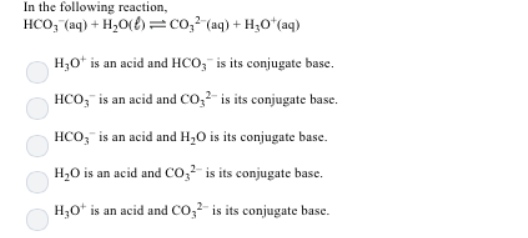# Problem: In the following reaction, HCO3- (aq) + H2O (l) ⇌ CO32- (aq) + H3O+ (aq) a. H3O+ is an acid and HCO3- is its conjugate base. b. HCO3- is an acid and CO32- is its conjugate base. c. HCO3- is an acid and H2O is its conjugate base. d. H2O is an acid and CO32- is its conjugate base. e. H3O+ is an acid and CO32- is its conjugate base.

###### FREE Expert Solution
88% (243 ratings)###### Problem Details

In the following reaction,

HCO3- (aq) + H2O (l) ⇌ CO32- (aq) + H3O+ (aq)

a. H3O+ is an acid and HCO3- is its conjugate base.

b. HCO3- is an acid and CO32- is its conjugate base.

c. HCO3- is an acid and H2O is its conjugate base.

d. H2O is an acid and CO32- is its conjugate base.

e. H3O+ is an acid and CO32- is its conjugate base.Frequently Asked Questions

What scientific concept do you need to know in order to solve this problem?

Our tutors have indicated that to solve this problem you will need to apply the Conjugate Acids and Bases concept. If you need more Conjugate Acids and Bases practice, you can also practice Conjugate Acids and Bases practice problems.

What professor is this problem relevant for?

Based on our data, we think this problem is relevant for Professor Bindell's class at UCF.